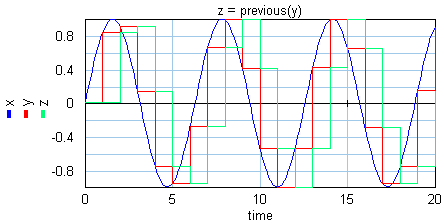﻿ 20-sim webhelp > Language Reference > Functions > Discrete > previous

# previous

## Syntax

y = previous(x,init);

## Description

The previous function delays the input signal x and holds it for one sample interval. It is equivalent to the z-1 discrete time operator.

The init argument is the first value for y at t=0 {s}.## Examples

x = sin(time*0.3);

y = sample(x);

z = previous(y);

u = hold(z);

## Limitations

x and y will become discrete signals. 20-sim will automatically detect the existence of discrete signals. Each chain of discrete signals will be assigned a specific sample time. You can set this sample time to any desired value in the Simulator (choose Properties, Simulation and Discrete System).